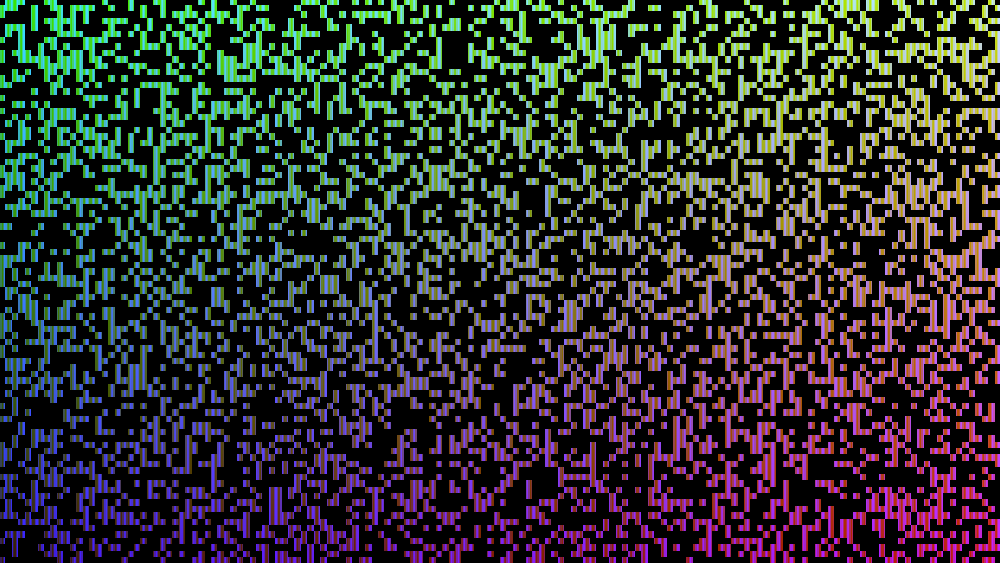# Pixel transition

A pixel transition effect where the screen fills with pixels. Use it on an overlay ColorRect or Sprite.

``````// Pixel transition shader

float rand(vec2 co){
return fract(sin(dot(co.xy ,vec2(12.9898,96.233))) * 43758.5453);
}
uniform float time = 1.0;
void fragment()
{
vec2 iResolution = 1.0 / SCREEN_PIXEL_SIZE;
vec2 uv = FRAGCOORD.xy / iResolution.xy;
float resolution = 5.0;
vec2 lowresxy = vec2(
floor(FRAGCOORD.x / resolution),
floor(FRAGCOORD.y / resolution)
);

if(sin(time) > rand(lowresxy)){
COLOR = vec4(uv,0.5+0.5*sin(5.0 * FRAGCOORD.x),1.0);
}else{
COLOR = vec4(0.0,0.0,0.0,0.0);
// change to COLOR = vec4(0.0,0.0,0.0,1.0); to make black pixels
}
}``````
###### Tags
blend, pixelate, transition### Lines Screen Transition

Subscribe
Notify of1 Comment
Inline Feedbacks